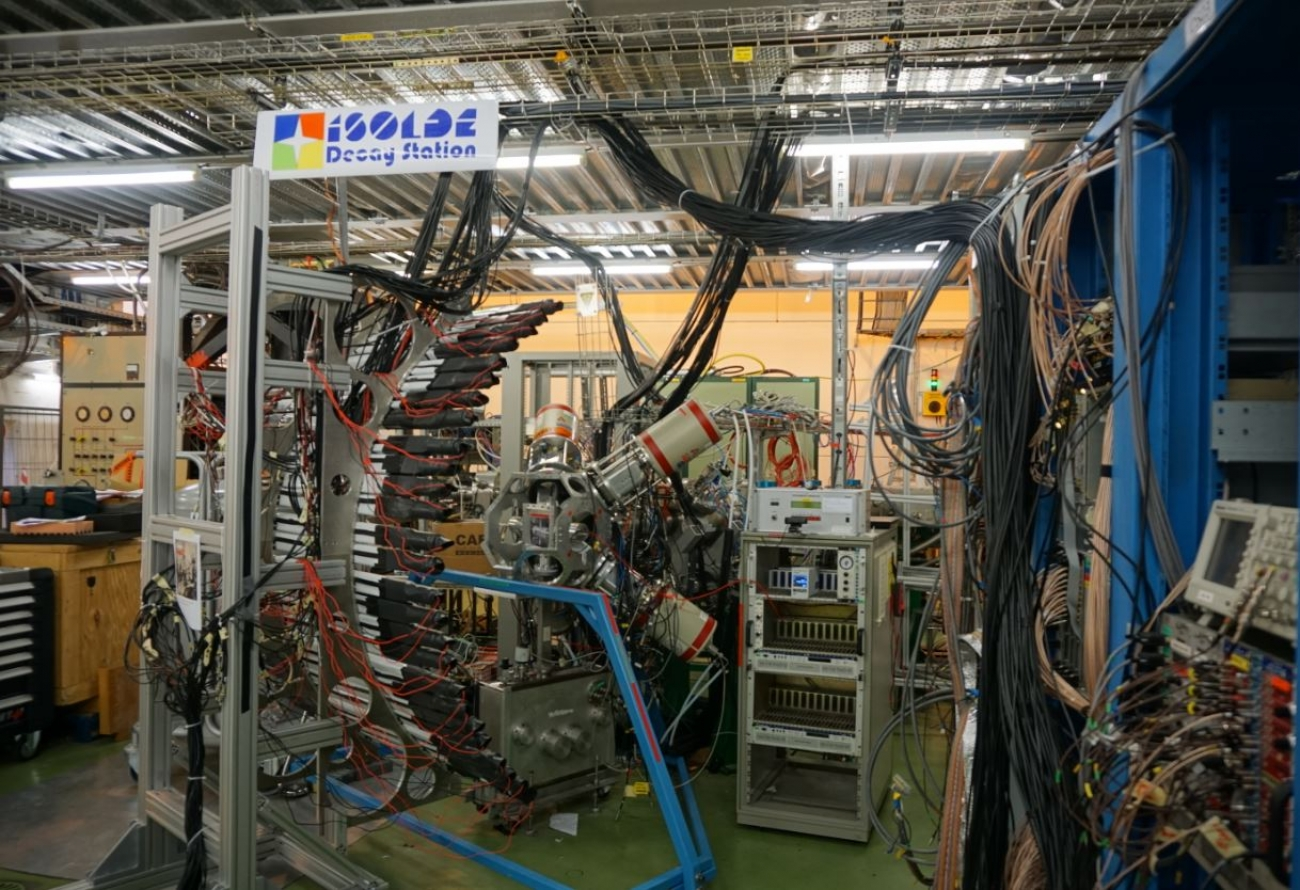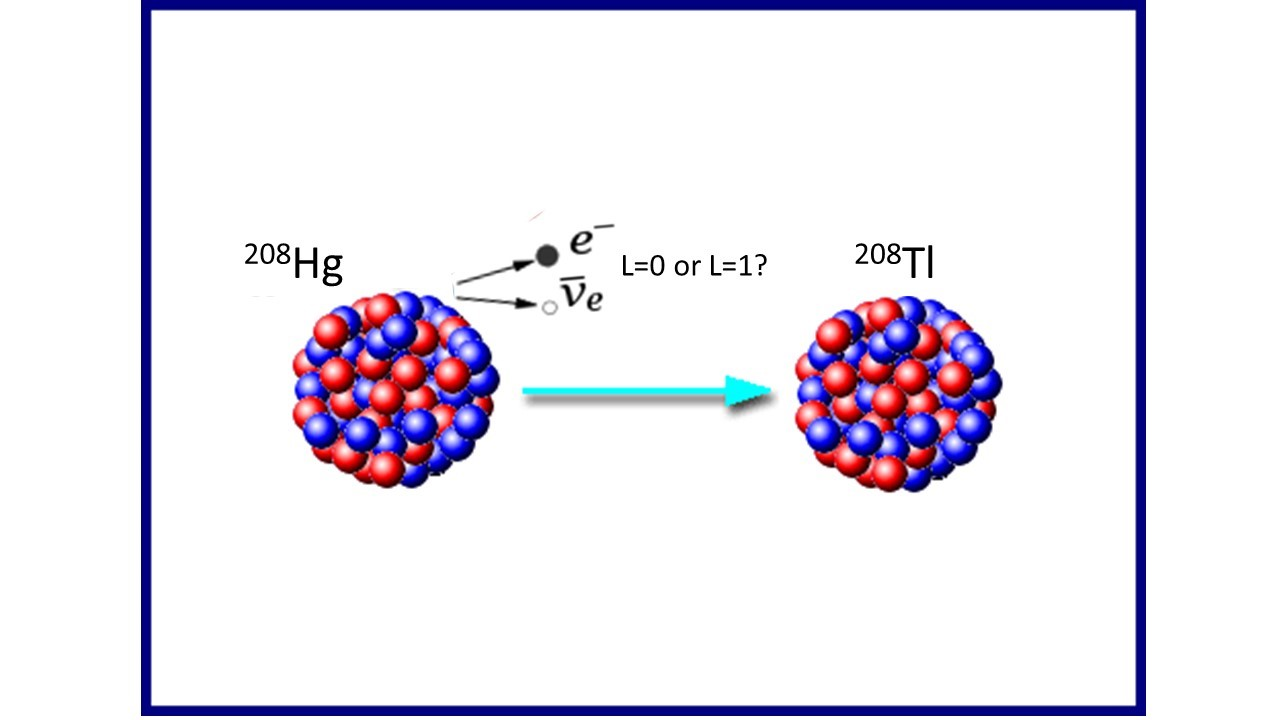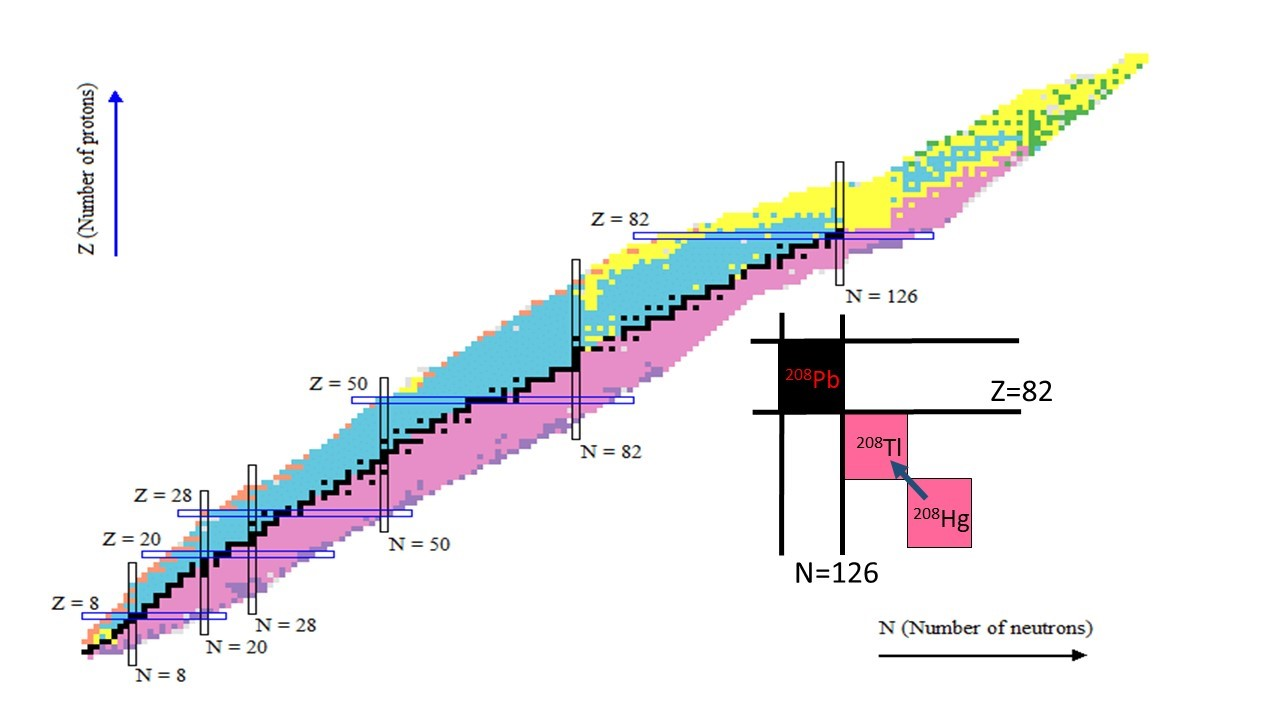# Secrets of beta decay unraveled at ISOLDEErnest Rutherford, in 1899, separated radioactive emissions into two types: alpha and beta. Becquerel, in 1900, measured the mass-to-charge ratio of beta particles and identified them as electrons. The theory of β decay was developed by Fermi in 1934. However, even now predicting the half-life of beta decaying nuclei is extremely challenging.

In beta decay an electron (or positron) is emitted from the atomic nucleus.  The energy spectrum of the beta particle is continuous, which led to the prediction of the neutrino, emitted together with the beta particle. The β decay is classified as allowed (if the orbital angular momentum carried by the β particle and neutrino is L =0), first-forbidden (L =1) and so on (see figure 1). The so-called forbidden transitions are hindered, but not completely suppressed. The selection rules for allowed β decay require no parity change between the initial (decaying) and final (populated) states. In contrast, in first-forbidden decay there is a parity change.Figure 1: Beta decay is categorised, based on the orbital angular momentum of the beta particle, as allowed (L=0), first forbidden (L=1) etc.

As the name suggests, the vast majority of beta decay is via allowed transitions. But there are regions of nuclei where forbidden decays are expected to compete and even dominate. One of them, the region of heavy nuclei with large neutron-to-proton ratio, influences the nucleosynthesis of heavy elements in neutron-star mergers. Since these nuclei cannot be produced on Earth, we have to rely on theoretical prediction. However, calculating forbidden decays is notoriously difficult.

The beta decay of 208Hg into 208Tl was studied at ISOLDE at CERN. The parent neutron-rich nucleus 208Hg was produced by bombarding a molten lead target with protons provided by the PS booster. The separated 208Hg nuclei were implanted in a tape, and gamma rays following their beta decay recorded at the ISOLDE Decay Station. These provided information on the quantum states of 208Tl.

An ideal atomic nucleus to study the competition between allowed and forbidden β decay should have a small number of both positive and negative parity levels which could be populated in beta decay, and they should have simple and well-understood wave functions.

Shell structure naturally arises for interacting multi-particle quantum systems. The concept has been successfully used in atomic physics, nuclear physics, and for metallic clusters. Nuclei are different from the other systems, in that both protons and neutrons form their own shells separately. The beauty of the shell model is that only the additional valence nucleons have to be considered, while the core containing magic numbers of protons and neutrons can be assumed to be inert. Therefore, quantum excitations in nuclei in the vicinity of doubly magic numbers have well defined wave functions. In addition, the 208Tl nucleus (with one proton-hole and one neutron-particle outside the closed shells) is expected to have a small number of both positive and negative parity low-energy states. Therefore, the beta decay of 208Hg into 208Tl provides an ideal testing ground for the study of the competition between allowed and forbidden beta decay (see figure 2).Figure 2: The nuclide chart with proton numbers (elements) on the y axis and neutron number on the x axis. The colour code indicates decay modes: black are stable nuclei, purple and blue are β-and β+ decaying, respectively. The magic number for protons (Z) and neutrons (N) are indicated. The inset is a simplified magnification showing the focus of the present study.

The ISOLDE experiment revealed that three negative parity excited states in 208Tl were populated directly in beta decay. In contrast none of the positive parity states were populated. This latter can be understood considering not that the atomic nucleus transforms in beta decay, but a single proton decays into a neutron. Therefore, the properties of the individual particle matter. The measured first-forbidden decay probabilities are in line with systematic uncertainties, however at present these could not be reliably calculated.

In addition, since 208Tl contains one proton and one neutron valence particles, the experiment provided the first test of the effective proton-neutron interaction in this region. This is essential for predicting the properties of nuclei with more valence particles, within the quadrant defined by proton number Z<82 and neutron number N>126.

These ISOLDE results focused on the fundamental characteristics of beta decay provide important constraints on theoretical models addressing the competition between allowed and first-forbidden β decays, which in turn are important for the detailed understanding of the nucleosynthesis of heavy elements produced in neutron start mergers.